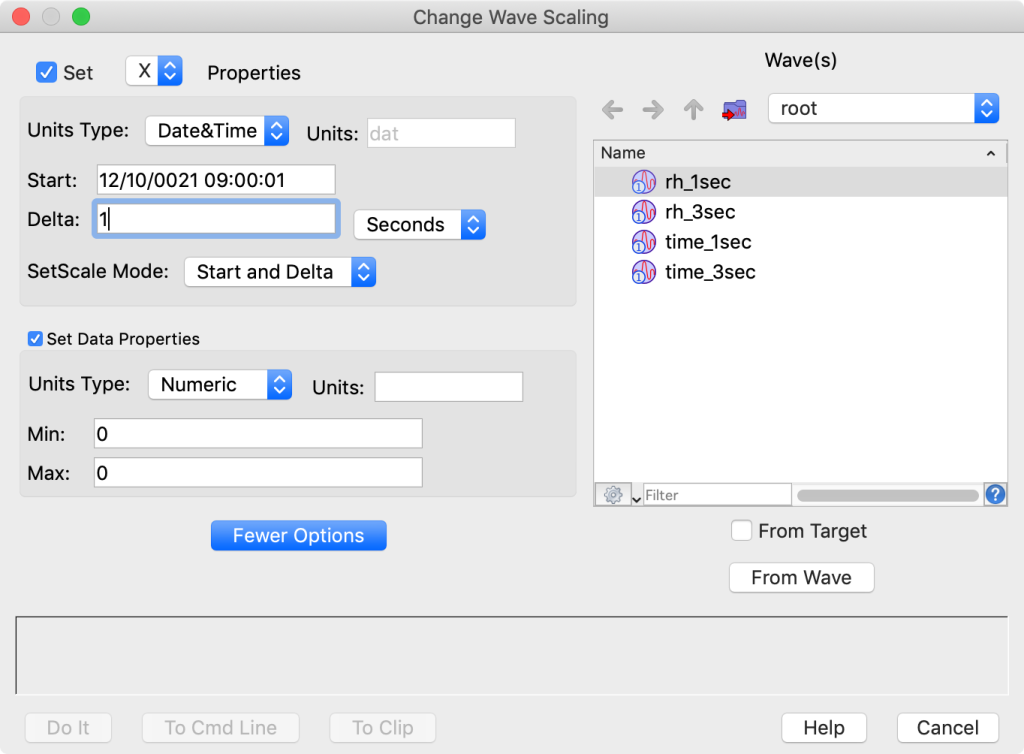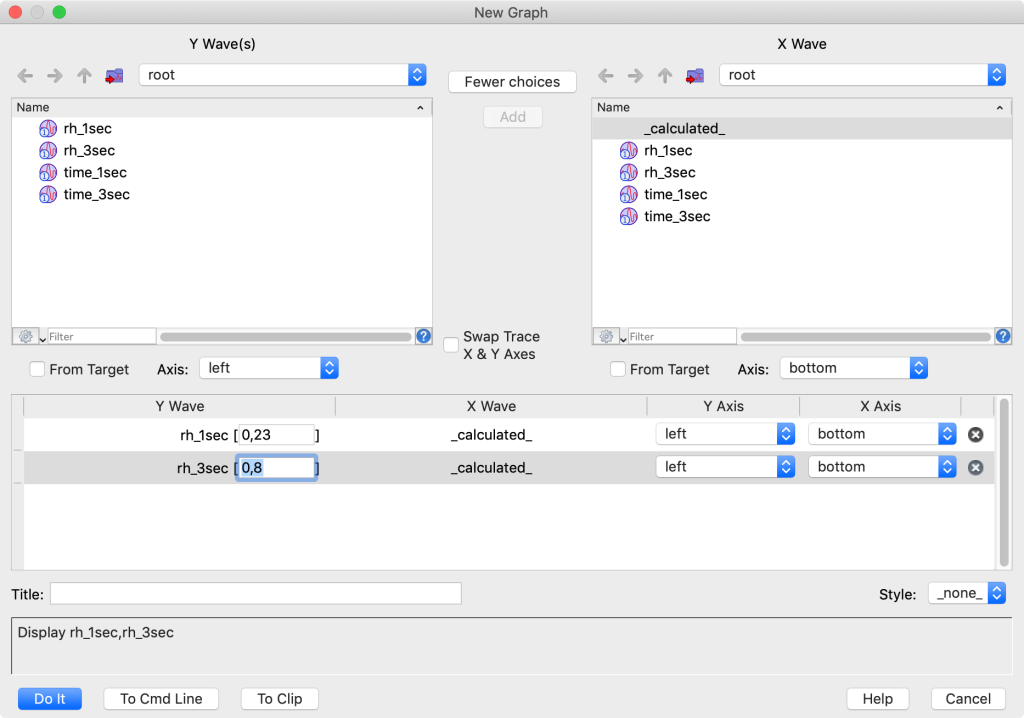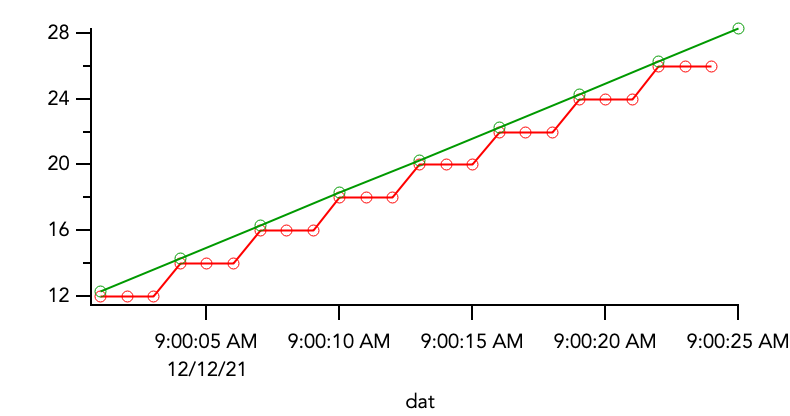# Wave ScalingThis is a concept I just can't get a handle on. I've looked at the help for SetScale and have spent a couple of hours looking at different forum posts on scaling and still don't get it. I need to understand what is going on when scaling happens. I've attached a test experiment with test data and wrote some thoughts down in a notebook. Thanks in advance for any help that the community can provide.

S

Hi,

The concept of wave scaling is to embed the x points into the y data set.  This allows you to have to keep track of only the y data.  Take the cue from the name waves, a regular pattern.  For your date, you have two waves of y data rh_1sec and rh_3sec.  The crux of wave scaling says for rh_1sec:  the first point is at 12/10/21 09:00:01 and every point afterwards is 1 second later.  So we really need only keep track of that starting time and the interval in point number.  So the time at any point in the y data is given by

12/10/21 09:00:01 + n*(1 sec)

Similarly for the rh_3sec

12/10/21 09:00:01 + n*(3 sec)In the history window you see.

`•SetScale/P x -59391932399,1,"dat", rh_1sec`
`Which translates to set x and the first number is the starting point (time in secs relative to jan 1,1904), the second number is the increment(1 sec) which is the /P flag denotes, "dat" signals to Igor to plot this value as a date. And the last is the y data to act on.`

Now when you want to deal with the waves, you can just use the y data. Notice for the x _calculated_ is selected.And the resulting graph. Igor knows it is a date and the points are spaced as intended.There is more power than just making graphing have a few less data sets to keep track of.  For example you can get a value for which you do not have a an actual point and Igor will interpolate based on the point scaling.

Andy

Thanks Andy, this is good stuff. So now can i use this to create RH waves that are the same length? In my real application I am calibrating RH senors (the 1 sec data) against an RH standard (the 3 sec data). I have hours worth of data at different RH values, with 8 different sensors,similar in trend, to the red plot in the previous diagram. Each plateau being about 15 minutes in duration. So when using the cursors and wavestats, I want to select an area on the plateau with the cursors to average and without moving the cursors do wavestats on all of the sensors. This doesn't work unless the waves are all the same length. So, I think what i need is for all of my data on the same time scale with the same interval. So can scaling make my 3sec wave longer by interploting between points or my 1sec data waves shorter by "removing" points? I'm not a fan of adding data. So basically I think i would want to select the value from the 1 sec data that corresponds to the 3 sec time stamp. Does this make sense? Thanks again for any help.

Scott

You can use the xcsr function to get the x value of the point at which a cursor is placed. So you can use this function to get the x values of the start and end time over which you want to evaluate.

WaveStats has a /R flag, and you can provide either x values using /R=(startX,endX) or you can provide x indexes using /R=[startP,endP]. In your case, you can use /R=(startX,endX) using the beginning and end x values you got from the xcsr calls.

You can write a simple function that gets the x range you want to use for your average and then finds the average for each wave on the graph over that range.

Here are some commands you can execute on Igor's command line to bring up related help topics:

DisplayHelpTopic "Programming With Cursors"
DisplayHelpTopic "Programming With Trace Names"

This command will bring up an example function that you could start with:

`DisplayHelpTopic "Wave Accessed Via Wave Reference Function"`

You would need to change the call to mean into a call to WaveStats and add in the xcsr calls before you get into the loop.

Also, before you get too far into this, I suggest that you do at least the first half of the guided tour if you haven't done so already. You can use the Help->Getting Started menu to get there. That will give you a high level overview of many of the important concepts you need to use Igor efficiently (including wave scaling).

I'm not a complete newb with IGOR, although at times like this that it seems so. At one point I did go through the getting started stuff... anyway getting back to my problem. When we Wave scale we are assigning a time to each y-wave point using the start time and delta in the "change Wave Scaling" dialog. Correct? Not using the time wave associated with the original data. So when I scale my rh_1sec with a start time that matches the original data and set the delta to 3 my 24 data points are spread out over 72 seconds instead of the original 24. Correct? How do I make the rh_1sec and the rh_3 sec wave the same length. Data points in the shorter wave would have to be added(interpolated) or points removed from the longer wave? Perhaps wave scaling is not what I should be doing here....I probably should be doing what aclight suggested: "WaveStats has a /R flag, and you can provide either x values using /R=(startX,endX) or you can provide x indexes using /R=[startP,endP]. In your case, you can use /R=(startX,endX) using the beginning and end x values you got from the xcsr calls." By using the start and end x values, not indices, to calculate the mean values is the way to go?

Hi,

If you need the waves to be the same length, you will need to interpolate.

The simplest way to do this is to take advantage of the wave scaling.

For example:

rh_3 is the wave with 3 sec intervals

rh_1 is the wave with 1 second interval

and we want a wave with the equivalent of 1 sec intervals but from the 3 sec wave.

Duplicate the rh_1 to new wave where we will put the the interpolated data using duplicate will also bring the wave scaling

duplicate rh_1, rh_3_1sec

then

rh_3_1sec = rh_3(x)  uses all the x values, the point scaling from the new wave) and returns the value from rh_3 at that point interpolating if necessary.

Andy

In reply to by ssmith911

ssmith911 wrote:

Perhaps wave scaling is not what I should be doing here....I probably should be doing what aclight suggested: "WaveStats has a /R flag, and you can provide either x values using /R=(startX,endX) or you can provide x indexes using /R=[startP,endP]. In your case, you can use /R=(startX,endX) using the beginning and end x values you got from the xcsr calls." By using the start and end x values, not indices, to calculate the mean values is the way to go?

My use of WaveStats with /R=(startX,endX) assumes that you are using wave scaling. Otherwise it would be equivalent to /R=[startP,endP].

I would like to thank everyone for their comments and responses. I now understand the concept of scaling and how to use the "change wave scaling" dialog to embed x values , either numeric or time based, into the y wave. Once this was done I was able to place one set of cursors on one of my 8 plots and use the xcsr with the wavestats function to get averages on all of the plots without having to move the cursors. Thanks for the help.

On that one, I have a (trivial) question as well:

I have several data waves from an instrument loaded into IGOR. I have changed the wave scaling using the "Change Wave Scaling" dialog.
All waves have a dd.mm.yyyy hh:mm:ss.000 (miliseconds) Format. All waves have a different start time. This start date and time I have set in the "Change Wave Scaling" dialog.

What is the easiest way to find out at which is the exact date and time of the first data point of each individual wave, when I come back in a week or so?

Thanks a lot

Hello Andy,

thanks - correct, I was aware of that one. However, there is two issues with this:

1) The start point is shown in seconds since 1904; I require dd.mm.yyyy hh:mm:ss.000

2) The number of the start point is abbreviated like 3.73962e+09; this is an approximate number. I require the exact start point

Thanks and regards

Another possibility is to make a table:

Edit/W=(5,45,798,739) junk.xy
ModifyTable format(Point)=1,format(junk.x)=8,showFracSeconds(junk.x)=1,width(junk.x)=150

Note the "showFracSeconds" keyword applied to the X column in the table. With that keyword, Igor shows the fractional part of the time.